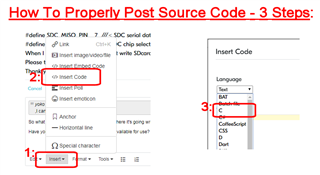# uart communication

enum state_t{idle=0,AA,Cal_lnth,Evt_Data_clt,Cmd_Data_clt,Ack_Data_clt,cal_Check_sum};
enum events_t{aa=0x55,event=0x55,BB=0xbb,timeout,ack=0x33,cmd=0xcc,length};
uint16_t time_out=0;
uint8_t packet,Index=0,Event,ps;
struct State_Matrix
{
enum state_t  Current_state;
enum events_t Current_event;
enum state_t     Next_state;

S_Matrix[]  = { {       idle   ,        aa,  AA            },
{         AA   ,     event,  Evt_Data_clt  },
{         AA   ,       cmd,  Cmd_Data_clt  },
{         AA   ,       ack,  Ack_Data_clt  },
{Evt_Data_clt  ,    length,  cal_Check_sum },
{Ack_Data_clt  ,    length,  cal_Check_sum },
{Cmd_Data_clt  ,    length,  cal_Check_sum },
{          AA  ,    timeout,     idle      },
{Cal_lnth      ,    timeout,     idle      },
{Evt_Data_clt  ,    timeout,     idle      },
{Ack_Data_clt  ,    timeout,     idle      },
{Cmd_Data_clt  ,    timeout,     idle      },

enum events_t f1();
enum events_t f2();
enum events_t f3();
enum events_t f4();
enum events_t f5();
enum events_t f6();

struct State_function

{
char name;
enum events_t (*func)();
};
Function[]  = {      {"    idle     ",       &f1 },
{"     AA      ",       &f2 },
{"Cal_lnth     ",       &f3 },
{"Evt_Data_clt ",       &f4 },
{"Cmd_Data_clt ",       &f5 },
{"Ack_Data_clt ",       &f6 },

};

void f1(uint8_t i,uint8_t a[])
{
while(packet[i]!=aa)
i++;
ps = AA;
}
void  f2(uint8_t i,uint8_t *ptr)
{
LPC_TIM0->TCR = 1;
if(packet[i] == 0x55)
*ptr = Evt_Data_clt;
else if(packet[i] == 0x33)
*ptr = Ack_Data_clt;
else if(packet[i] == 0x55)
*ptr = Cmd_Data_clt
else
;
}
void  f3()
{
LPC_TIM0->TCR = 1;

}
enum events_t f4()
{
LPC_TIM0->TCR = 1;
if(length>16)
ps = cal_Check_sum;
}
enum events_t f5()
{
LPC_TIM0->TCR = 1;
if(length>14)
ps = cal_Check_sum;
}
enum events_t f6()
{
LPC_TIM0->TCR = 1;
if(length>16)
ps = cal_Check_sum;
}

int UART0_IRQHandler()
{
LPC_TIM0->TCR = 0;
packet[Index] = LPC_UART0->RBR;
if(Index==0)
Function[Index+1].func(Index,packet);

}
void TIMER0_IRQHandler()
{
LPC_TIM0->TCR = 2;//TIMER0_IRQHandler,precounter are reset
Event  = timeout;
ps  = idle;
}
int main()
{
LPC_GPIO3->FIODIR3 = 0xff;
LPC_GPIO3->FIOPIN3 = 0x00;
LPC_SC->PCONP  = (1<<3|3<<1);//poweron uart0 in pconp register at bit3 and timer0
LPC_SC->PCLKSEL0  = 0<<6;//peripheral clock select for uart0 in pclksel0 registers 6-7 bits
LPC_UART0->LCR  = 1<<7;//divisor latch enabled in lcr register
LPC_UART0->DLL  = 0xa1;//register contains lsb of divisor
LPC_UART0->DLM  = 0x00;//register contains msb of divisor
LPC_UART0->FCR  = 1;//enable fifo in fcr
LPC_PINCON->PINSEL0 = (1<<4)|(1<<6);//ENABLED UART0 TX & RX OF GPIO
LPC_UART0->LCR  = 0<<7;//dlab=0
LPC_UART0->LCR  = 3|(0<<2)|(0<<3);
LPC_GPIO3->FIODIR3 = 0xff;
LPC_GPIO3->FIOPIN3 = 0x00;
LPC_UART0->IER  = 1;
NVIC->ISER  = 1<<5;
LPC_SC->PCLKSEL0  = 0<<2;//peripherical=cclk is selected for timer0
LPC_TIM0->PR  = 0;//precount register set to zero
LPC_TIM0->MR0  = 0xa2c3;
LPC_TIM0->MCR  = 3;

while(1)
{

}
Parents
••Children
No data
More questions in this forum

10 views
0 replies
Started
by

47 views

384 views
4 replies
Latest
by